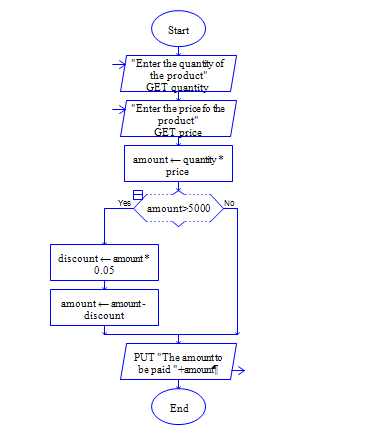# Java Program to calculate discount of 5% for purchase above 5000

Get price and quantity of a product, calculate bill amount and calculate 5% discount for the billamount 5000 and above.

Sample Input 1:

10 400

Sample Output 1:

4000

Sample Input 2:

20 500

Sample Output 2:

9500

#### Flow Chart DesignStrongly recommended to Solve it on your own, Don't directly go to the solution given below.

public class Hello { public static void main(String args[]) { //Write your code here } }

#### Program or Solution

``` import java.util.*; class Discount { public static void main(String args[]) { double quantity,price,amount,discount; Scanner sc=new Scanner(System.in); System.out.println("Enter The Price Of The Product:"); price=sc.nextDouble(); System.out.println("Enter The No Of Quantity:"); quantity=sc.nextDouble(); amount=quantity*price; if(amount>5000) { discount=amount*0.05; amount=amount-discount; } System.out.println("The Total Amount is:"+amount); } } ```

#### Program Explanation

1. Get two inputs quantity and price from user using scanner class

2. Calculate amount by using the expression amount = quantity * price

3. Check whether amount is greater than 5000 using if statement.

if amount is greater than 5000 then calculate discount and amount using the expressions

3a.   discount = amount * 0.05

3b.   amount = amount - discount

4. print amount using system.out.println

3a and 3b will be executed if 3 returns true else those two won't be executed.